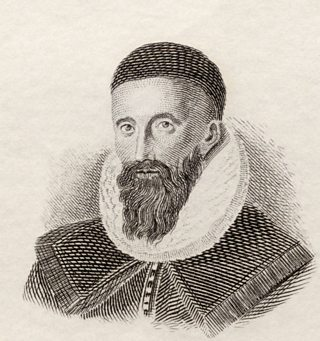# Multiplication – Napier’s methodCredit: Ken Welsh/Bridgeman
Portrait of Scottish mathematician, John Napier (1550 – 1617)

This method uses a grid to multiply numbers.

Multiplying a two-digit number with another two-digit number would require abygrid, for example.

Multiplyingwould require abygrid.

Follow the steps below to see how Napier’s method is used to calculate.

• The first step is to draw abygrid.
• The second step is to draw a diagonal in each box. The diagonal line separates the tens and the units. Always write the tens above the diagonal line in each box.
• Start by multiplyingandto fill the left box on the top row.
•• Writeandto show that there are no tens.
• Now multiplyandto fill the right box on the top row.
•• Next, multiplyandto fill the left box on the bottom row.
•• Remember to put theabove the diagonal.
• Complete the grid by multiplyingandto fill the right box on the bottom row.
•After completing the grid, add the columns along the diagonals, starting at the bottom-right. We sometimes need to carry over from one diagonal to the next. To get the answer, read the totals down the left and to the right.Question

Use Napier’s method to calculate.

Draw abox and follow the guidelines in the previous example.Did you notice that there’s no need to carry over in this example?